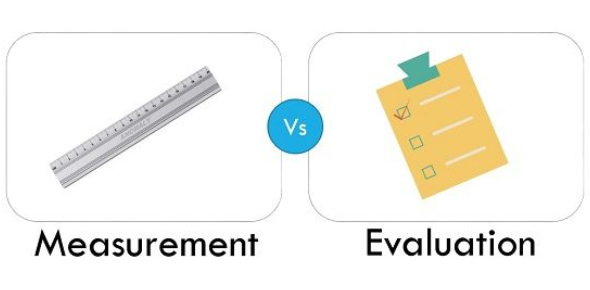# Measurement Evaluation MCQ Quiz Questions And Answers

20 Questions | Attempts: 10152
ShareSettingsDo you know about measurement and evaluation, and what are their purposes? Try out these questions and answers asked here in this quiz and assess your understanding level. Evaluation measures how and to what extent the program activities have met the objectives. Both the terms (evaluation and measurement) are used to measure pupils' achievement and motivate their learning. The below quiz contains a few basic questions related to these two analysis terms. Shall we begin the test now? Best of luck, dear friend!

• 1.
Involves the collection, organization and analysis of numerical data.
• A.

Measurement

• B.

Statistics

• C.

Assessment

• D.

Test

• 2.
Process of assigning a number to a performance or an attribute of a person.
• A.

Measurement

• B.

Evaluation

• C.

Assessment

• D.

Test

• 3.
Instrument used to make a particular measurement.
• A.

Statistic

• B.

Measurement

• C.

Evaluation

• D.

Test

• 4.
Process of measuring, evaluating, identifying, and prescribing.   Using results to identify performance and problems, and then prescribing a solution.
• A.

Assessment

• B.

Measurement

• C.

Test

• D.

Evaluation

• 5.
Usually numerical and is the result of a measurement.
• A.

Sample

• B.

Variable

• C.

Test

• D.

Data

• 6.
Trait of a characteristic that can assume more than one value.
• A.

Discrete Data

• B.

Ungrounded data

• C.

Population

• D.

Variable

• 7.
Part of subgroup of the given population in which every member has an equal chance of being included in the sample.
• A.

Random Sample

• B.

Ungrouped Data

• C.

Grouped Data

• D.

Continuous Data

• 8.
A ration scale includes a interval scale with a true 0 point?
• A.

True

• B.

False

• C.

Somewhat true

• D.

Somewhat false

• 9.
Sample is a part or subgroup of the given population.
• A.

True

• B.

False

• C.

Somewhat true

• D.

Somewhat false

• 10.
Measure that can have any value within a certain range.
• A.

Random Sample

• B.

Continuous Data

• C.

Discrete Data

• D.

Grouped Data

• 11.
Measures that can have ONLY seperate values?
• A.

Discrete Data

• B.

Sample Data

• C.

Random Sample

• D.

Variable Data

• 12.
Measures not arranged in any meaningful manner?
• A.

Discrete Data

• B.

Random Variable

• C.

Grouped Data

• D.

Ungrouped Data

• 13.
Measures are arranged in some meaningful manner.
• A.

Discrete Data

• B.

Variable Data

• C.

Grouped Data

• D.

Ungrouped Data

• 14.
Provides information about the order of variable and uses equal units of measurement.
• A.

Ordinal Scale

• B.

Interval Scale

• C.

Ratio Scale

• D.

Nominal Scale

• 15.
Score that represents the exact midle or the 50% of the group.
• A.

Mean

• B.

Median

• C.

Range

• D.

Mode

• 16.
It describes the middle characteristics of a distribution of scores.
• A.

Mean

• B.

Mode

• C.

Median

• D.

All of the above

• 17.
The scores that occur the most?
• A.

Mode

• B.

Range

• C.

Median

• D.

None

• 18.
Needs for statistics in the physical education, health and AT fields.
• A.

Motivation

• B.

Diagnosis

• C.

Testing

• D.

Examination

• E.

Classification

• F.

Achievement

• G.

Data Recording

• H.

Prediction

• 19.
Meaningful intrepretation by letting you figure out the scores Spread or scatter.
• A.

Standard deviation

• B.

Measures of variability

• C.

Measures of changeability

• D.

None

• 20.
Considers the deviation each score is away from the mean of the distribution.
• A.

Mean Deviation

• B.

Mean

• C.

Mode

• D.

Median

## Related TopicsBack to top
×

Wait!
Here's an interesting quiz for you.